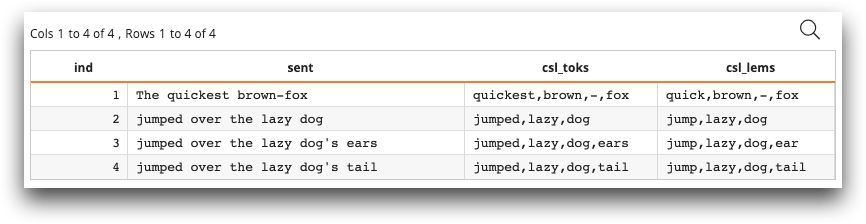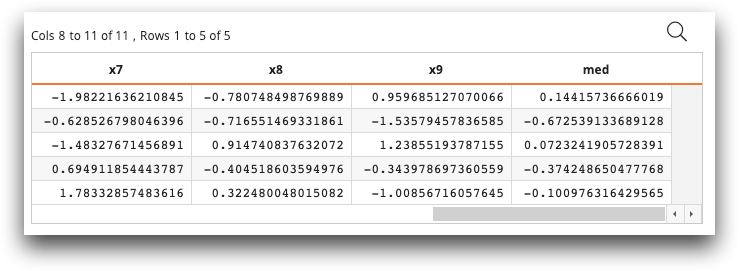# Defining user and group functions

You can use the Macro Language tag `<resource>` to define a resource for Macro Language using Python code. You can also use `<def_ufun>` and `<def_gfun>` to define your own Python functions within the Macro Language Workshop.

The `<resource>` tag consists of definitions relevant for a particular language. The `<def_ufun>` tag allows you to create your own functions that work in a row-by-row or scalar context, while the `<def_gfun>` tag allows you to create your own group or tabulation functions.

Note: See `<resource>`, `<def_ufun>`, and `<def_gfun>` in the 1010data Reference Manual for a detailed description of these tags, including syntax and a list of attributes.

## Example: Defining natural language processing functions

The following example defines a Macro Language resource called `"nlp"`, written in Python, and using the open source library spaCy. Then we use `<def_ufun>` to define the functions `clean_tokenizer_to_csl` and `clean_lemmatizer_to_csl`. Each function takes a string as an argument and returns a string. The functions themselves are written in Python. Finally, we create a table in Macro Language and create two columns with `<willbe>``csl_toks` and `csl_lems`—that make use of the new functions that tokenize and lemmatize the string in `sent`, respectively.

```<library>
<resource for="python" name="nlp">
<![CDATA[
import spacy
]]>
</resource>
<resource for="mdb" name="nlp">
<def_ufun name="clean_tokenizer_to_csl" args="x" types="s(s)">
<code language_="python">
<![CDATA[
r = [','.join([x.text for x in nlp(s) if not x.is_stop]) for s in x]
]]>
</code>
</def_ufun>
<def_ufun name="clean_lemmatizer_to_csl" args="x" types="s(s)">
<code language_="python">
<![CDATA[
r = [','.join([x.lemma_ for x in nlp(s) if not x.is_stop]) for s in x]
]]>
</code>
</def_ufun>
</resource>
</library>
<table cols="ind,sent">
1,"The quickest brown-fox"
2,"jumped over the lazy dog"
3,"jumped over the lazy dog's ears"
4,"jumped over the lazy dog's tail"
</table>
<willbe name="csl_toks" value="nlp.clean_tokenizer_to_csl(sent)"/>
<willbe name="csl_lems" value="nlp.clean_lemmatizer_to_csl(sent)"/>```

The resulting table looks like the following:## Example: Quantile function across a row of data

The following example defines a quantile function in Python and applies it across each row of data. First, we define two functions: `r_quantile` and `mk_lst_nms`. `r_quantile` takes as its arguments `w`, a list of columns on which to apply the quantile, and `p`, a float that contains the quantile, and returns a float, which is the value of the arrays at the given quantile. `mk_lst_nms` takes as its arguments `s`, a string, and `n`, an integer, and returns a string, which is a comma-separated list of the names of the column data. Then in Python, we create a table with 5 rows and 10 columns, populated with random data. Finally, we use `<willbe>` and the newly created `r_quantile` function, containing a quantile of .5, to create the `med` column. The `med` column contains the median of the values of each row.

```<base table="default.lonely"/>
<library>
<def_ufun name="r_quantile" args="w;p" types="f(Ln;f)">
<code language_="python">
<![CDATA[
# w is initially a list of numpy arrays
# first turn into numpy matrix
# then take quantile along rows.
r = np.quantile(np.array(w),p,axis = 0)
]]>
</code>
</def_ufun>
<def_ufun name="mk_num_nms" args="s;n" types="s(s;i)">
<code language_="python">
<![CDATA[
r = [s + str(i) for i in range(0,n)]
]]>
</code>
</def_ufun>
</library>
<code language_="python">
<![CDATA[
nr, nc = 5,10
# note that I'm creating a table, NOT a matrix
dat = [np.random.randn(nr) for i in range(0,nc)]
nms = ['x' + str(i) for i in range(0,nc)]
md = ten.MetaData().from_arrays(dat, names = nms, labels = nms)
ops = ten.rebase(dat,md)
]]>
</code>
<willbe name="med" value="r_quantile({mk_num_nms('x';10)};0.5)"/>```

The resulting table looks similar to the following:The last column, med, contains the .5 quantile (median) of each row.